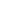| lesson material | material summary | questions and answers | definitions | types and examples | other information | materi pelajaran | ringkasan materi | pertanyaan dan jawaban | definisi | jenis-jenis dan contoh-contoh | informasi lainnya |

# Learning PHP: 7 Types of Operators in PHP that must be knownDo I have to be good at math to be a programmer?
No, because to learn basic programming ...
... we don't have to be good at math.
Even my friend who majored in mathematics also couldn't coding. Although they also learn programming languages ​​like Pascal and Matlab.
Knowing the basics of mathematics is enough to prepare for learning programming.
One of them is the science of number and logic operations.
In programming, we are familiar with operators.
Operators are symbols used to carry out operations on a value and variable.
There are 6 types of operators in PHP programming that we must know:
1. Arithmetic Operators;
2. Assignment Operator or Assignment ;
3. Operator Increment & Decrement ;
4. Relational operator or comparison;
5. Logic Operator;
6. Bitwise operator;
7. and Ternary Operators.
Let's discuss them one by one…

## 1. Arithmetic Operators

Arithmetic operators are operators to carry out arithmetic operations.
The arithmetic operator consists of:
Operator NameSymbol
Addition`+`
Reduction`-`
Multiplication`*`
Appointment`**`
Division`/`
Remaining Share`%`
Example:
``````<?php

\$a = 5;
\$b = 2;

// penjumlahan
\$c = \$a + \$b;
echo "\$a + \$b = \$c";
echo "<hr>";

// pengurangan
\$c = \$a - \$b;
echo "\$a - \$b = \$c";
echo "<hr>";

// Perkalian
\$c = \$a * \$b;
echo "\$a * \$b = \$c";
echo "<hr>";

// Pembagian
\$c = \$a / \$b;
echo "\$a / \$b = \$c";
echo "<hr>";

// Sisa bagi
\$c = \$a % \$b;
echo "\$a % \$b = \$c";
echo "<hr>";

// Pangkat
\$c = \$a ** \$b;
echo "\$a ** \$b = \$c";
echo "<hr>";

?>
``````
At first we have two variables, namely `\$a`and `\$b`with the initial values ​​as follows:
``````\$a = 5;
\$b = 2;
``````
Then we use the arithmetic operator to perform operations on these two values ​​or variables.
Then the results are stored in variables `\$c`.
Then the result: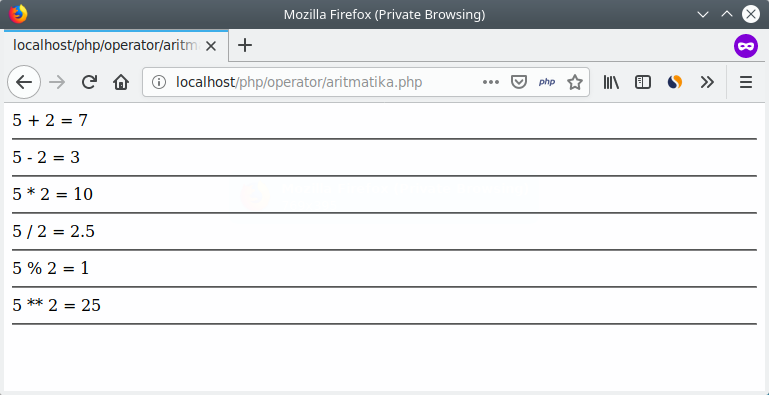O yes, note also the operator symbols that are used.
In mathematics, multiplication usually uses symbols `x`But in multiplication programming uses symbols `*`.
``````// salah
\$c = 7 x 4;

// benar
\$c = 7 * 4;
``````
Then for operators `%`(modulo), this is the operator to calculate the remainder for.
For example:
``````// kita punya 5
\$a = 5;

// lalu kita bagi dengan 2
\$c = \$a / 2;
``````
Then the variable `\$c`will be valuable `1`, because it is `5`divided into the `2`remainder `1`.
Let it be easier, try to imagine it like this:
You have `5`seed candy , then share it with your sister. To be fair, both get two seeds. Well, there are one remaining that hasn't been shared.
The rest is the result of modulo.

## 2. Assignment operator

The next operator that you must deal with is the assignment operator or assignment .
Yep! of course the name is predictable.
This operator is an operator to assign assignments to variables.
Usually used to fill in values.
Example:
``````\$a = 32;
``````
Saama with ( `=`) is an assignment operator to fill in the value.
Apart from being the same, there are also several assignment orpeators such as:
Operator NameSombol
Charging Value`=`
Filling and Addition`+=`
Charging and Reduction`-=`
Filling and Multiplication`*=`
Filling and Lifting`**=`
Filling and Distribution`/=`
Charging and Remaining for`%=`
Filling and stringing`.=`
What's the difference with an arithmetic operator?
The assignment operator is used to fill values ​​and also calculate with arithmetic operations. While the arithmetic operator only functions to count.
As an example:
``````\$speed = 83;

// ini opertor aritmatika
\$speed = \$speed + 10;

// maka nilai speed akan samadengan 83 + 10 = 93

// ini operator penugasan
\$speed += 10;

// sekarang nilai speed akan menjadi 93 + 10 = 103
``````
Output: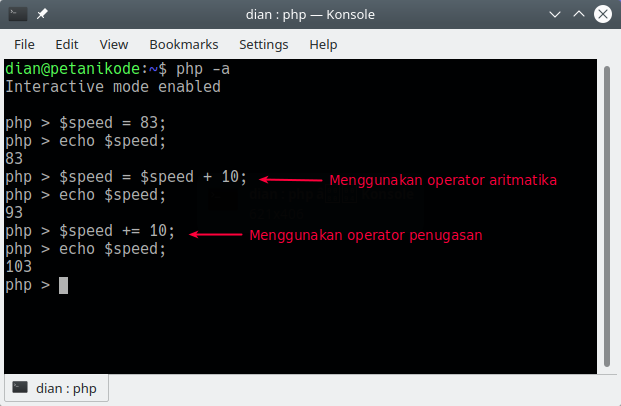Pay attention to this operation:
``````\$speed = \$speed + 10;
// dan
\$speed += 10;
``````
Both operations are the same operation. It's just that the top uses the arithmetic operator and the lower one uses the assignment operator.
In a way, the assignment operator is a simpler form of expression as above.
The use of assignment operators will often be found when making programs.

## 3. Opeartor Increment & Decrement

The increment and decrement operators are operators that are used to add `+1`(add one) and reduce `-1`(subtract one).
Opertor increments use symbols `++`, while decrements use symbols `--`.
Example:
``````\$score = 0;

\$score++;
\$score++;
\$score++;

echo \$score;
``````
Output: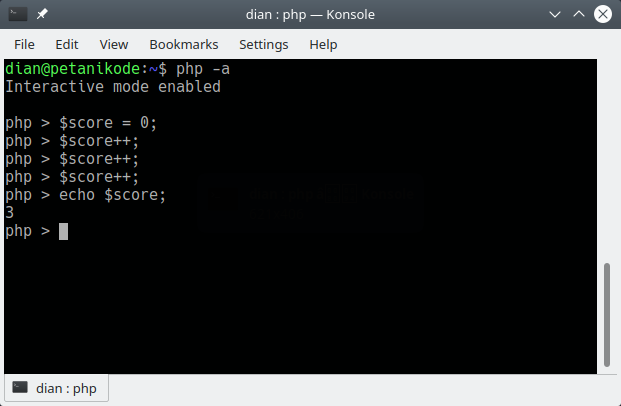Value `\$score`will be `3`, because we do increments of 3x.

## 4. Relationship Operators

A relation operator is an operator to compare two values.
The operating results of the relation operator will produce values ​​with boolean data types , namely `true`(true) and `false`(incorrect).
The following is a list of relation operators:
Operator NameSymbol
Greater than`>`
Smaller`<`
Together with`==` or `===`
Not equal to`!=` or `!==`
Bigger Same with`>=`
Smaller Same as`<=`
Let's try in the program: `relasi.php`
``````<?php

\$a = 6;
\$b = 2;

// menggunakan operator relasi lebih besar
\$c = \$a > \$b;
echo "\$a > \$b: \$c";
echo "<hr>";

// menggunakan operator relasi lebih kecil
\$c = \$a < \$b;
echo "\$a < \$b: \$c";
echo "<hr>";

// menggunakan operator relasi lebih sama dengan
\$c = \$a == \$b;
echo "\$a == \$b: \$c";
echo "<hr>";

// menggunakan operator relasi lebih tidak sama dengan
\$c = \$a != \$b;
echo "\$a != \$b: \$c";
echo "<hr>";

// menggunakan operator relasi lebih besar sama dengan
\$c = \$a >= \$b;
echo "\$a >= \$b: \$c";
echo "<hr>";

// menggunakan operator relasi lebih kecil sama dengan
\$c = \$a <= \$b;
echo "\$a <= \$b: \$c";
echo "<hr>";
``````
The result: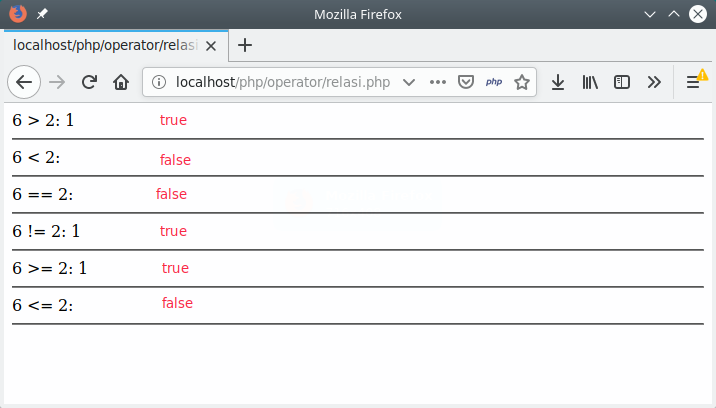Pay attention!
There we get the value `1`for `true`while `false`not displayed or `0`.
Is this wrong?
No, it is indeed like the nature of functions `echo`in PHP.
Values ​​with boolean data types are not usually displayed. Usually used to create conditions on branching .
Examples like this:
``````<?php
\$total_belanja = 150000;

if(\$total_belanja > 100000){
}
``````

## 5. Logic Operators

If you have studied mathematical logic, you will certainly not be familiar with this operator.
Logical operators are operators to perform logic operations such as `AND``OR`, and `NOT`.
Logical operators consist of:
Operator NameSymbol
Logic AND`&&`
Logic OR`||`
Negation / reverse / NOT`!`
Let's try in the program: `logika.php`
``````<?php

\$a = true;
\$b = false;

// variabel \$c akan bernilai false
\$c = \$a && \$b;
printf("%b && %b = %b", \$a,\$b,\$c);
echo "<hr>";

// variabel \$c akan bernilai true
\$c = \$a || \$b;
printf("%b || %b = %b", \$a,\$b,\$c);
echo "<hr>";

// variabel \$c akan bernilai false
\$c = !\$a;
printf("!%b = %b", \$a, \$c);
echo "<hr>";
``````
The result: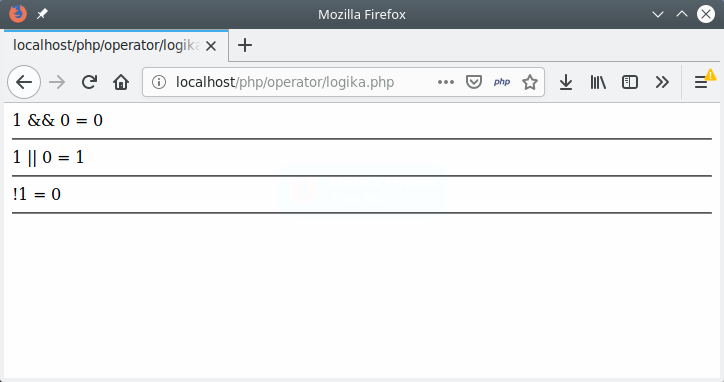Pay attention!
In the example above, we use a function `prinf()`to print formatting and print text.
But it will still display `1`for `true`and `0`for `false`.
The logic operator is the same as the relation operator, it will produce values ​​with boolean data types .
Note the results obtained when using operators `&&`(AND), `||`(OR), and `!`(NOT).
The operator `&&`will produce `true`if the left and right values ​​are worth `true`While the operator `||`will produce `false` when the left and right values ​​are worth `false`.
Try checking the logical laws AND, OR, and NOT.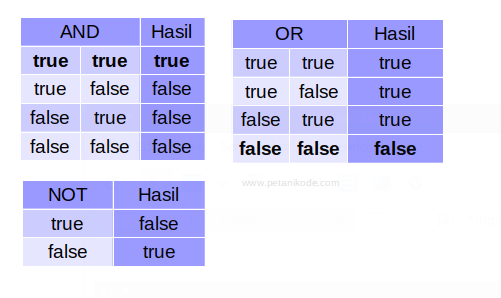## 6. Bitwise operator

The bitwise operator is an operator that is used for bit (binary) operations.
This operator consists of:
NameSymbol in Java
AND`&`
OR`|`
XOR`^`
Negation / reverse`~`
Left Shift`<<`
Right Shift`>>`
This operator applies for the data type `int``long``short``char`, and `byte`.
This operator will calculate from bit-to-bit.
For example, we have variables `a = 60`and `b = 13`.
When made in binary form, it will be like this:
``````a = 00111100
b = 00001101
``````
Then, do the bitwise operation
Operation AND
``````a = 00111100
b = 00001101
a & b = 00001100
``````
OR operation
``````a = 00111100
b = 00001101
a | b = 00111101
``````
XOR operation
``````a = 00111100
b = 00001101
a ^ b = 00110001
``````
NOT Opportunity
``````a = 00111100
~a  = 11000011
``````
The concept is almost the same as Opeartor Logic. The difference is, Bitwise is used for binary.
For more details, let's try in the program.
``````<?php

\$a = 60;
\$b = 13;

// bitwise AND
\$c = \$a & \$b;
echo "\$a & \$b = \$c";
echo "<br>";

// bitwise OR
\$c = \$a | \$b;
echo "\$a | \$b = \$c";
echo "<br>";

// bitwise XOR
\$c = \$a ^ \$b;
echo "\$a ^ \$b = \$c";
echo "<br>";

// Shift Left
\$c = \$a << \$b;
echo "\$a << \$b = \$c";
echo "<br>";

// Shift Right
\$c = \$a >> \$b;
echo "\$a >> \$b = \$c";
echo "<br>";
``````
The result: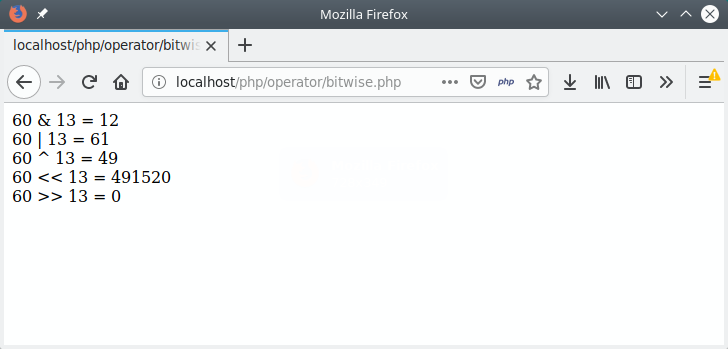## 7. Ternary Operators

The ternary operator is an operator to create a condition. The symbol used is a question mark ( `?`) and a colon ( `:`).In the example above, "You like me" is the question or condition that will be examined.
If the answer is correct, then yes. Otherwise it won't.
For more details, let's try ...
``````<?php

\$suka = true;

// menggunakan operator ternary
\$jawab = \$suka ? "iya": "tidak";

// menampilkan jawaban
echo \$jawab;

``````
The result: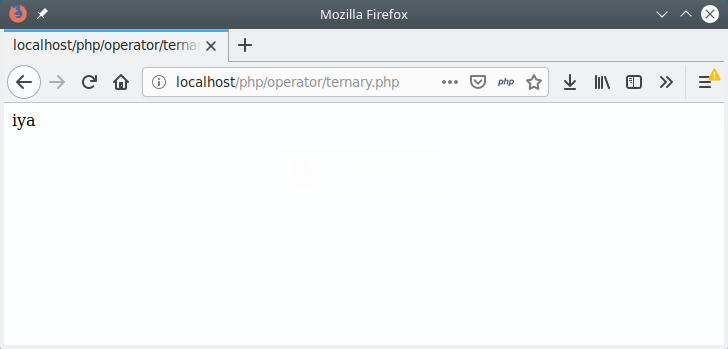Try to change the value of the variable `\$suka`to `false`, so the output will be `tidak`.

##### 0 Komentar untuk "Learning PHP: 7 Types of Operators in PHP that must be known"

Silahkan berkomentar sesuai artikel

Template By Kunci Dunia